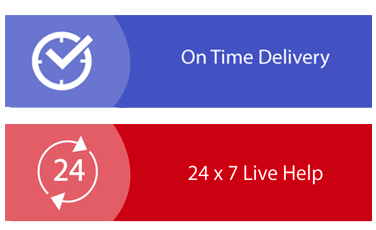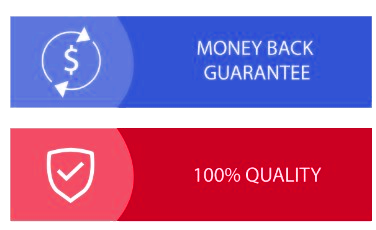× Limited Time Offer ! FLAT 20-40% off - Grab Deal Before It’s Gone. Order Now

## PROG2008 Computational Thinking Assignment Sample

Weight: 50% (40 hours)

In assignment 2, you have helped a real estate agent to gain some understanding of the market. The agent now wants you to help them set the indicative sale price for their new properties on the market. In this assignment, you will apply your knowledge in data analysis and modelling to build a regression model to predict the indicative sale price of a given property using the previous sale data.

### In particular, you will

• Apply multivariate analysis and appropriate visualization techniques to analyze the given dataset for the relationship between the sold price and other features of a property.

• Based on the analysis select one feature that can be used to predict the property indicative price. Justify your selection.

• Build a regression model to predict the indicative price from the selected feature.

• Train and validate the model using the given dataset and analyze the prediction power of the model. Discuss the result.

• Distinction students: propose a solution to improve the model accuracy.

• High Distinction students: implement the proposed solution to improve the model.

You will use Jupyter Notebook in this assignment to perform the required analyses, visualise data, and show the analysis results

## Solution

### Analysis Report for assignment help

The property sales prediction was done using a python programming language with Anaconda Framework using Jupyter Notebook IDE where the sales prediction dataset was explored at first. The libraries of python used for analysis and visualization were pandas, NumPy, matplotlib, seaborn, and sklearn for importing machine learning algorithms.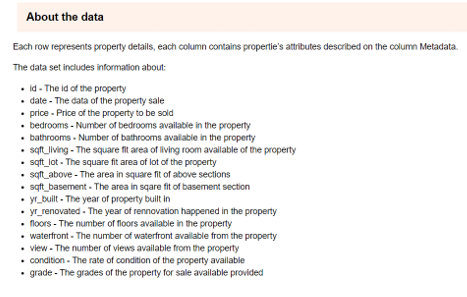The details about the data are thouroughly discussed in the notebook where each column details has been described about the property and sales.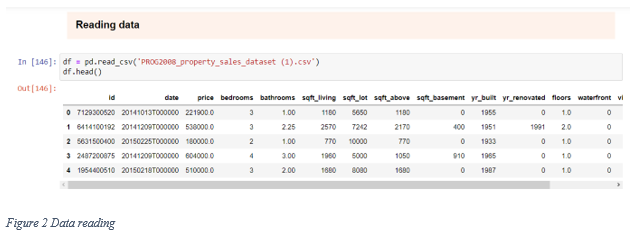The data reading was done using pandas where the information about the data was described.

Data processing including the column details, column information of data types, handling missing values and checking null values if present, and summary statistics of the data where the mean, deviation, maximum, count, etc. of the data are described.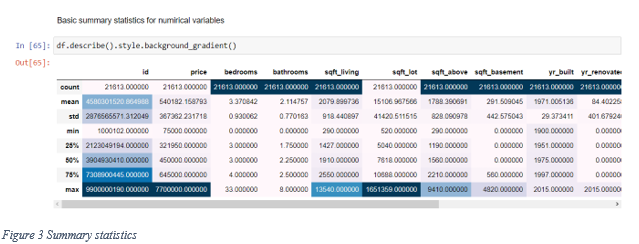### Data visualization

The data visualizations were done using matplotlib and seaborn library which are used for creating attractive and interactive graphs, plots, and charts in python. The different graphs from the data insights are described as communicating about the data containing visuals.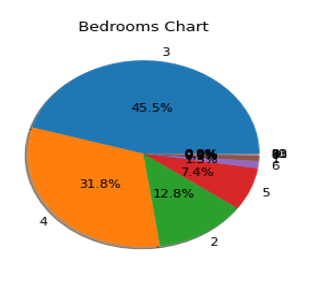The bar chart here describing the bedroom description count where the data containing 45.5% of property with bedrooms and the sales is also depended upon these factors.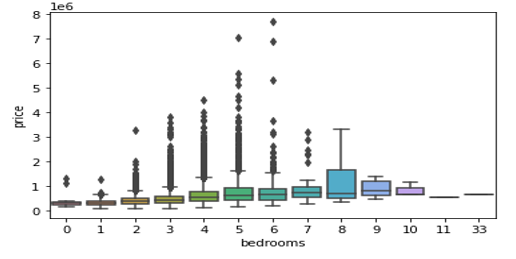Figure 5 Property sales price with bedroom availability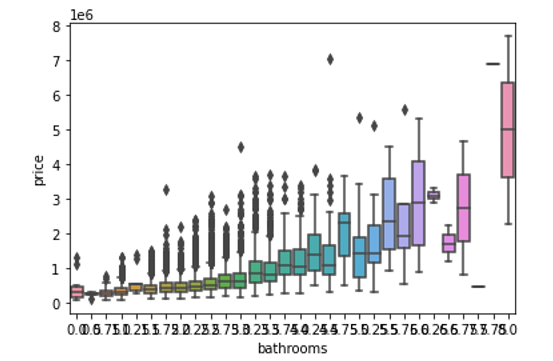Figure 6 Property sales price with bathroom availability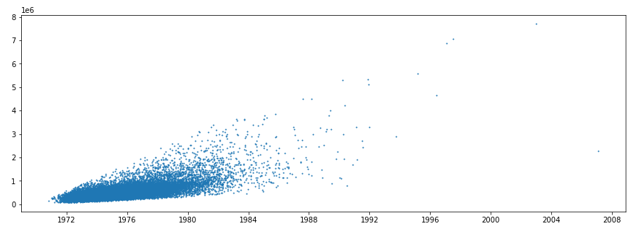Figure 7 Property sales price with square fit living availability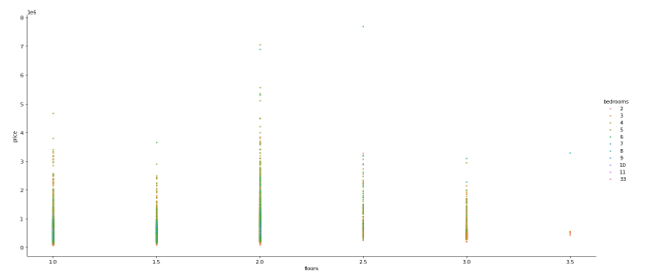Figure 8 Property sales price with floors availability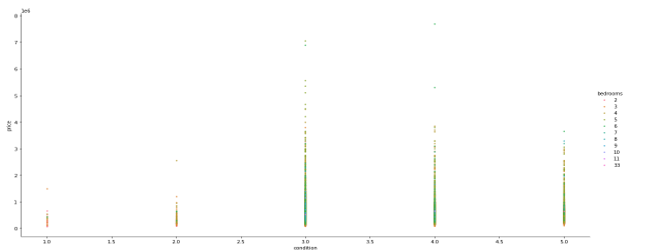Figure 9 Property sales price with condition availability

The sale prediction according to the property description is clearly described by the visualizations which describe the descriptive analysis of the data which represents the sales of the property according to the different infrastructure based happened in the past.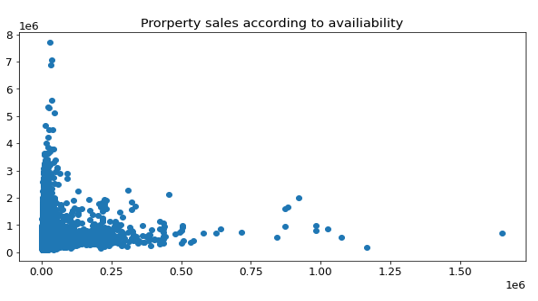Figure 10 Property sales price with space availability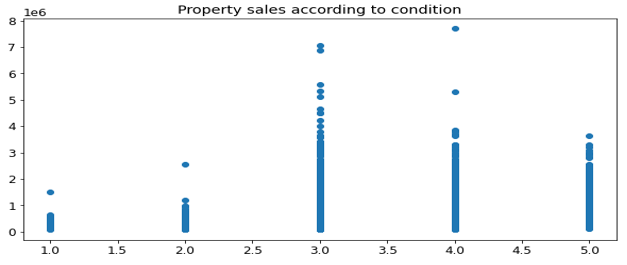Figure 11 Property sales price with condition availability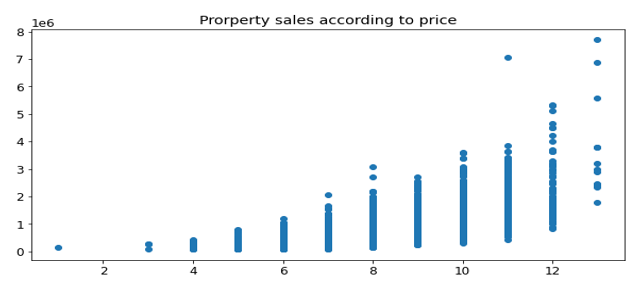Figure 12 Property sales price with grades availability

### Data modelling

The machine learning algorithm is applied to the dataset for the predictive analysis where the future prediction based on the descriptive analysis is done to look at whether the models are accurate according to the data and the accuracy of the model describes the predictive analysis rate to predict the property sales in the future.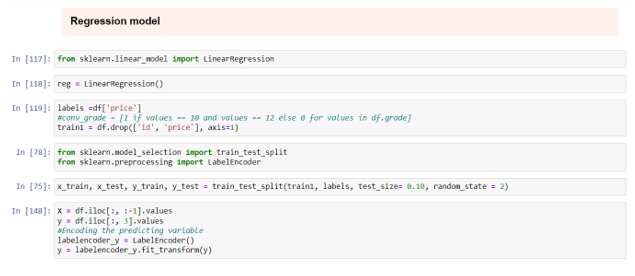Figure 13 Regression model

The algorithms are applied to the dataset by training the models by splitting the dataset into train and test split and then the trained and tested values are applied to the algorithms to calculate the score of the applied data.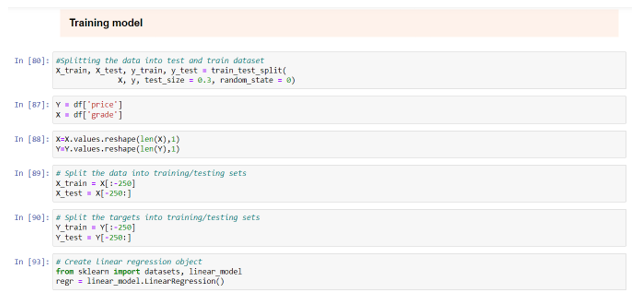Figure 14 Training of the model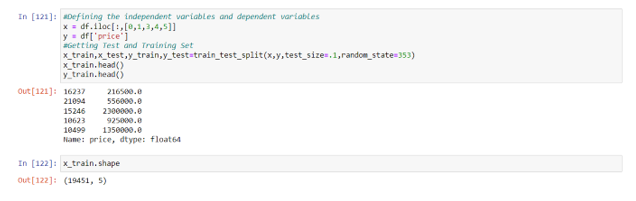Figure 15 Fitting regression model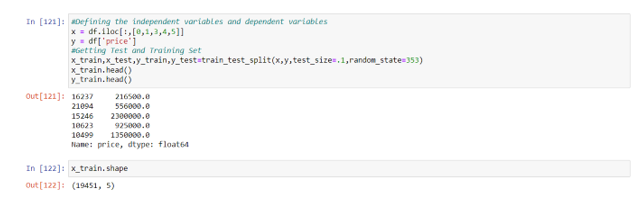Figure 16 Creating variables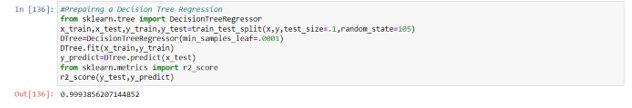Figure 17 Decision tree regression

The predictive analysis score of the linear regression model predicted 100% accuracy whereas the decision tree regression score comes to 99% accuracy which describes the property sales prediction as mostly accurate as assumed.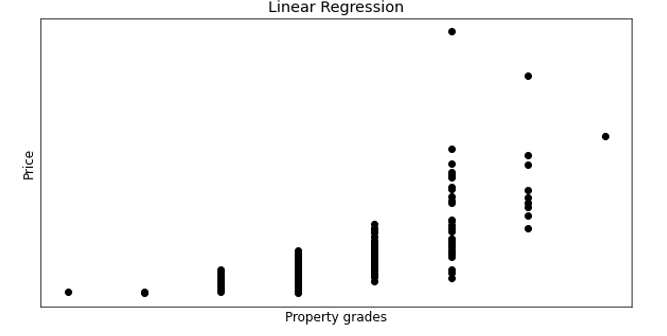Figure 18 Linear regression sales prediction with prices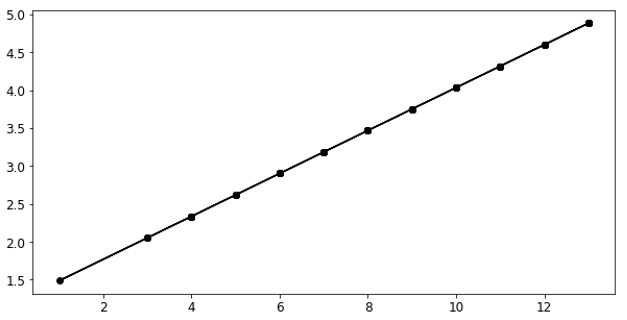Figure 19 Linearity of the model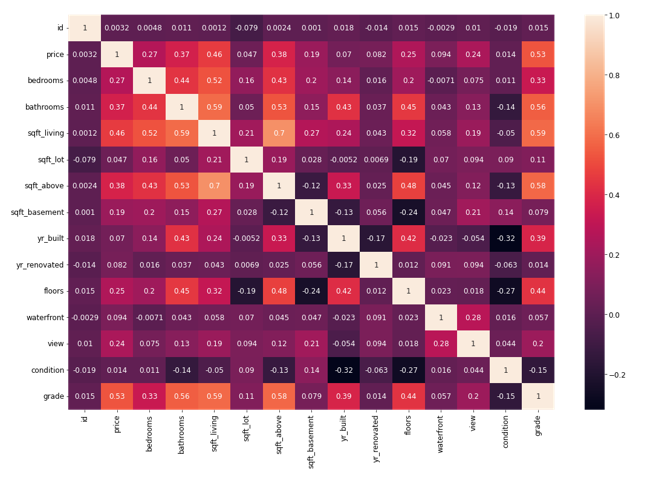Figure 20 Data Correlation heatmap

Fill the form to continue reading